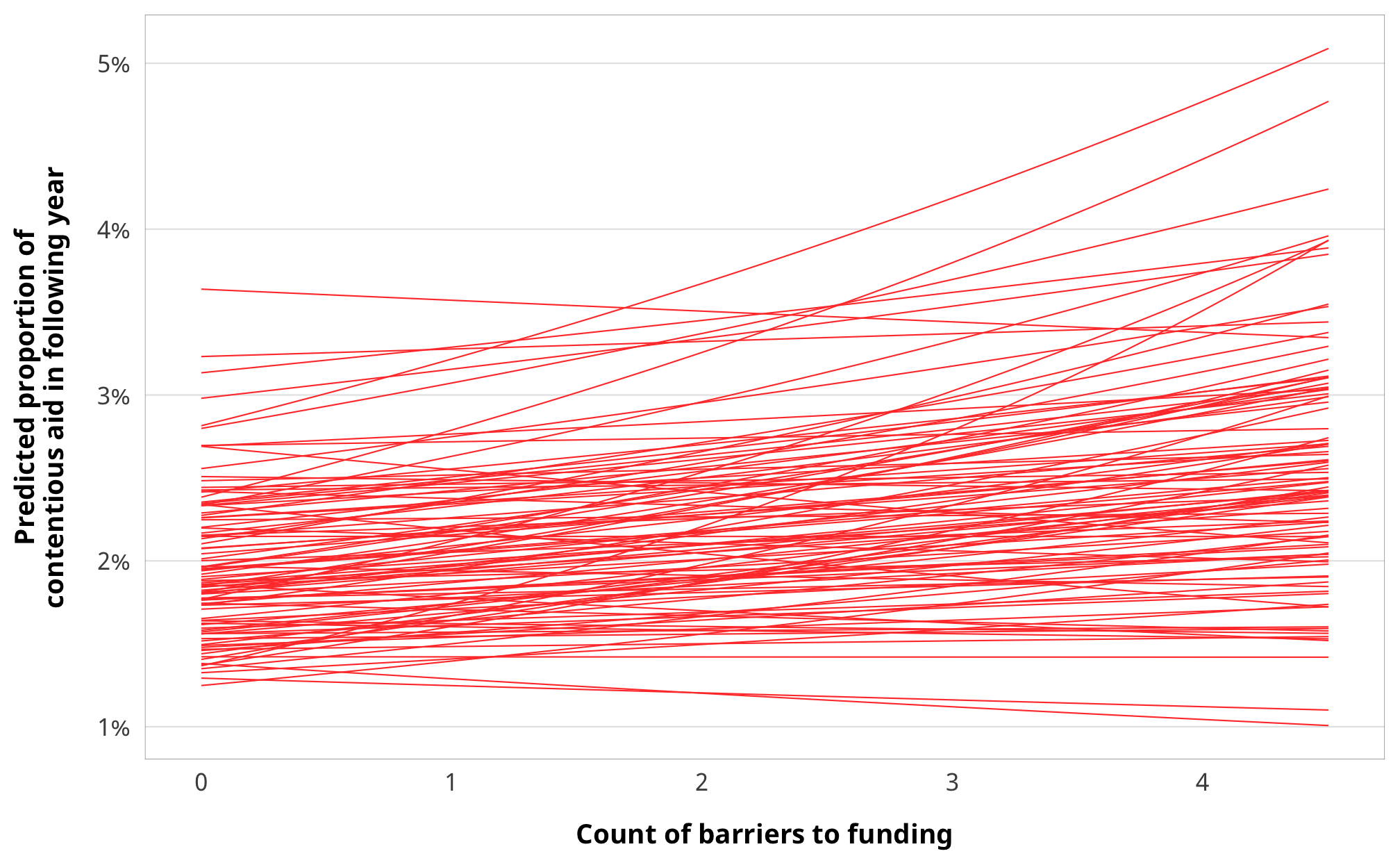library(tidyverse)
library(targets)
library(brms)
library(tidybayes)
library(bayesplot)
library(fixest)
library(broom)
library(broom.mixed)
library(modelsummary)
library(patchwork)
library(scales)
library(latex2exp)
library(kableExtra)
library(here)

color_scheme_set("viridisC")

my_seed <- 1234
set.seed(my_seed)

withr::with_dir(here::here(), {

# Data
df_country_aid_laws <- filter(df_country_aid, laws)

# Treatment models
m_purpose_treatment_entry, m_purpose_treatment_funding,
m_purpose_treatment_ccsi))

# IPTW data
df_purpose_iptw_entry, df_purpose_iptw_funding,
df_purpose_iptw_ccsi))

# Outcome models
m_purpose_outcome_entry, m_purpose_outcome_funding,
m_purpose_outcome_ccsi))

# Results tables
})

## Weight models

• Numerator is lagged treatment + non-varying confounders
• Denominator is lagged treatment + lagged outcome + time-varying confounders + non-varying confounders

$sw = \prod^t_{t = 1} \frac{\phi(T_{it} | T_{i, t-1}, C_i)}{\phi(T_{it} | T_{i, t-1}, D_{i, t-1}, C_i)}$

### Priors for weight models

We use generic weakly informative priors for our model parameters:

• Intercept: $$\mathcal{N} (0, 10)$$
• Coefficients: $$\mathcal{N} (0, 2.5)$$
• Sigma: $$\operatorname{Cauchy} (0, 1)$$
pri_int <- ggplot() +
stat_function(fun = dnorm, args = list(mean = 0, sd = 10),
geom = "area", fill = "grey80", color = "black") +
labs(x = TeX("\\textbf{Intercept (β_0)}")) +
annotate(geom = "label", x = 0, y = 0.01, label = "N(0, 10)", size = pts(9)) +
xlim(-40, 40) +
theme_donors(prior = TRUE)

pri_coef <- ggplot() +
stat_function(fun = dnorm, args = list(mean = 0, sd = 2.5),
geom = "area", fill = "grey80", color = "black") +
labs(x = TeX("\\textbf{Coefficients (β_x)}")) +
annotate(geom = "label", x = 0, y = 0.04, label = "N(0, 3)", size = pts(9)) +
xlim(-10, 10) +
theme_donors(prior = TRUE)

pri_sigma <- ggplot() +
stat_function(fun = dcauchy, args = list(location = 0, scale = 1),
geom = "area", fill = "grey80", color = "black") +
labs(x = "σ") +
annotate(geom = "label", x = 5, y = 0.08, label = "Cauchy(0, 1)", size = pts(9)) +
xlim(0, 10) +
theme_donors(prior = TRUE)

(pri_int + pri_coef + pri_sigma)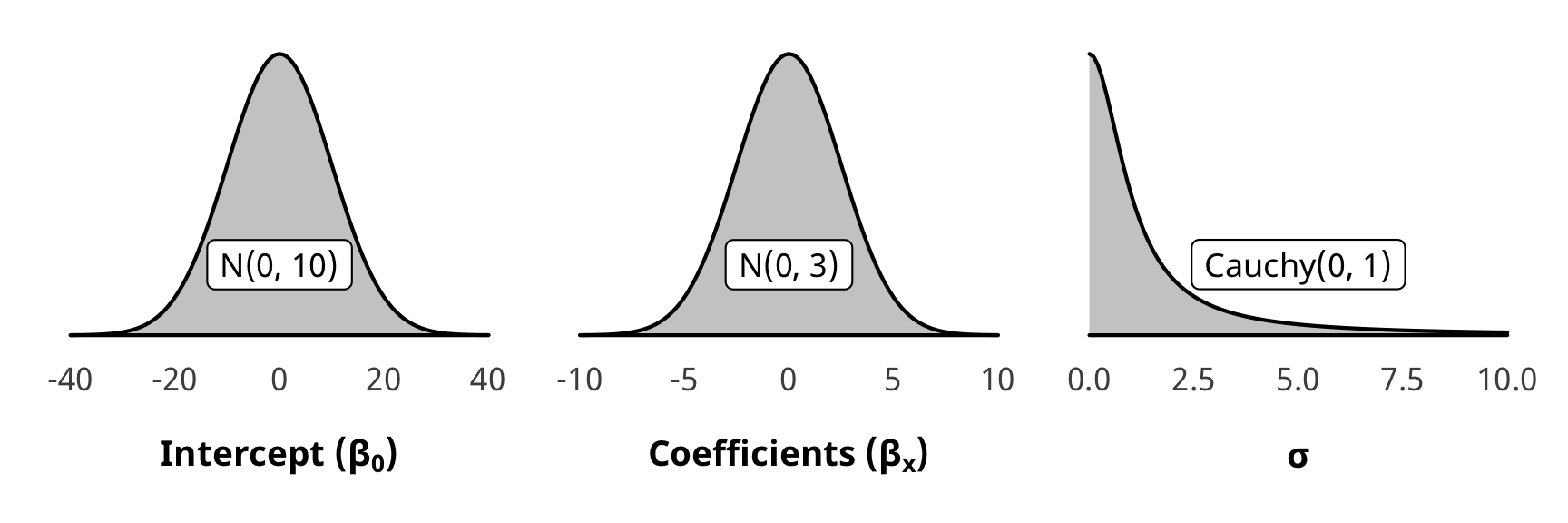### Check weight models

We check one of the denominator models for convergence and mixing and fit (not the numerator, since those models are the same as the ones in H1).

pp_check(m_purpose_treatment_total$model_denom, type = "dens_overlay", nsamples = 10) + theme_donors()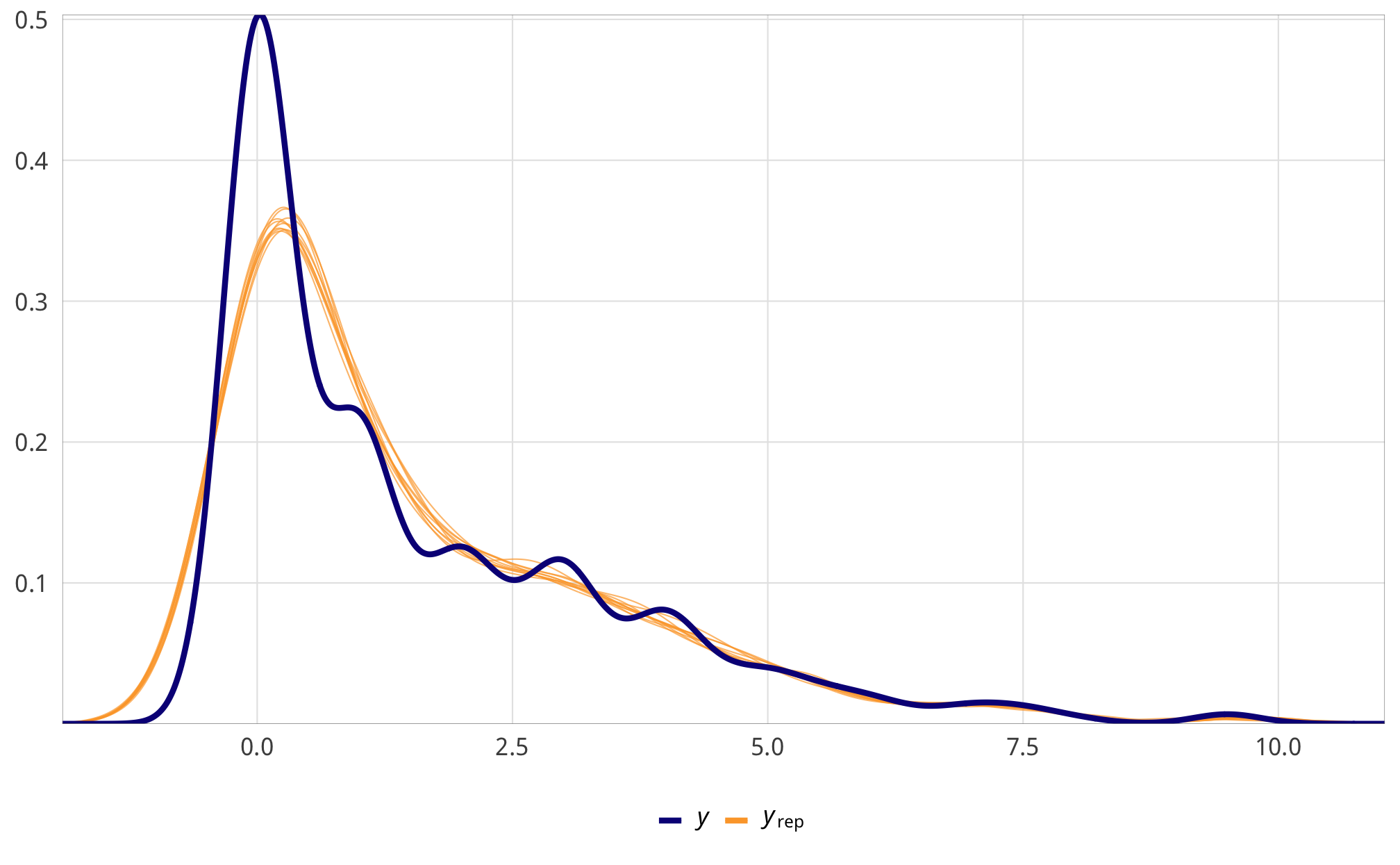m_purpose_treatment_total$model_denom %>%
select(-starts_with("r_gwcode"), -starts_with("z_"), -lp__, -iter) %>%
mcmc_trace() +
theme_donors() +
theme(legend.position = "right")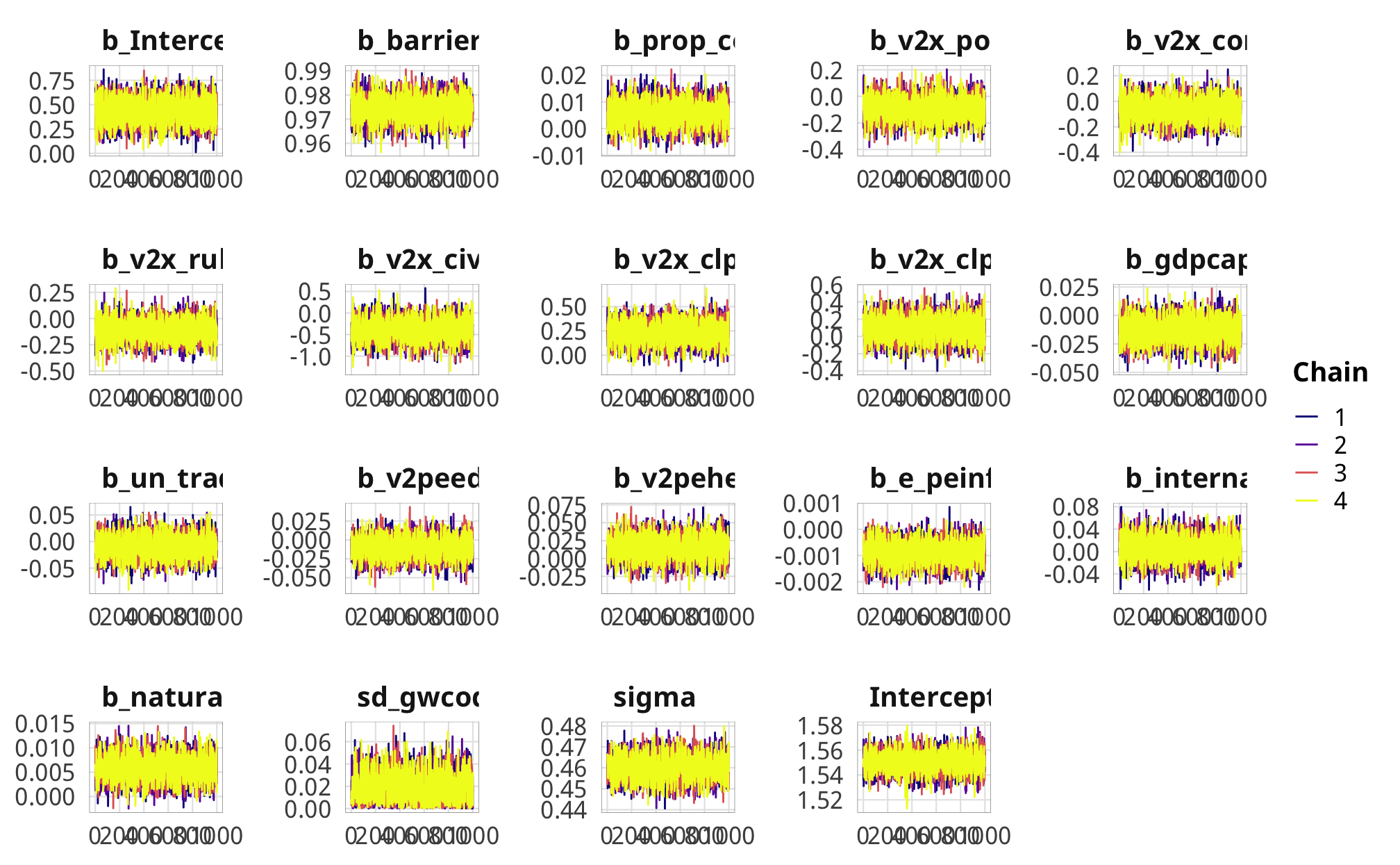### Check weights

IPTWs should generally have a mean of 1 and shouldn’t be too incredibly high.

#### Total ODA

Eh, some of these are high, but the mean and median are fine.

summary(df_purpose_iptw_total$iptw) ## Min. 1st Qu. Median Mean 3rd Qu. Max. ## 0.00259 0.85217 0.96672 1.14836 1.04919 19.68508 df_purpose_iptw_total %>% ggplot(aes(x = iptw)) + geom_histogram(binwidth = 0.5, boundary = 0) + theme_donors()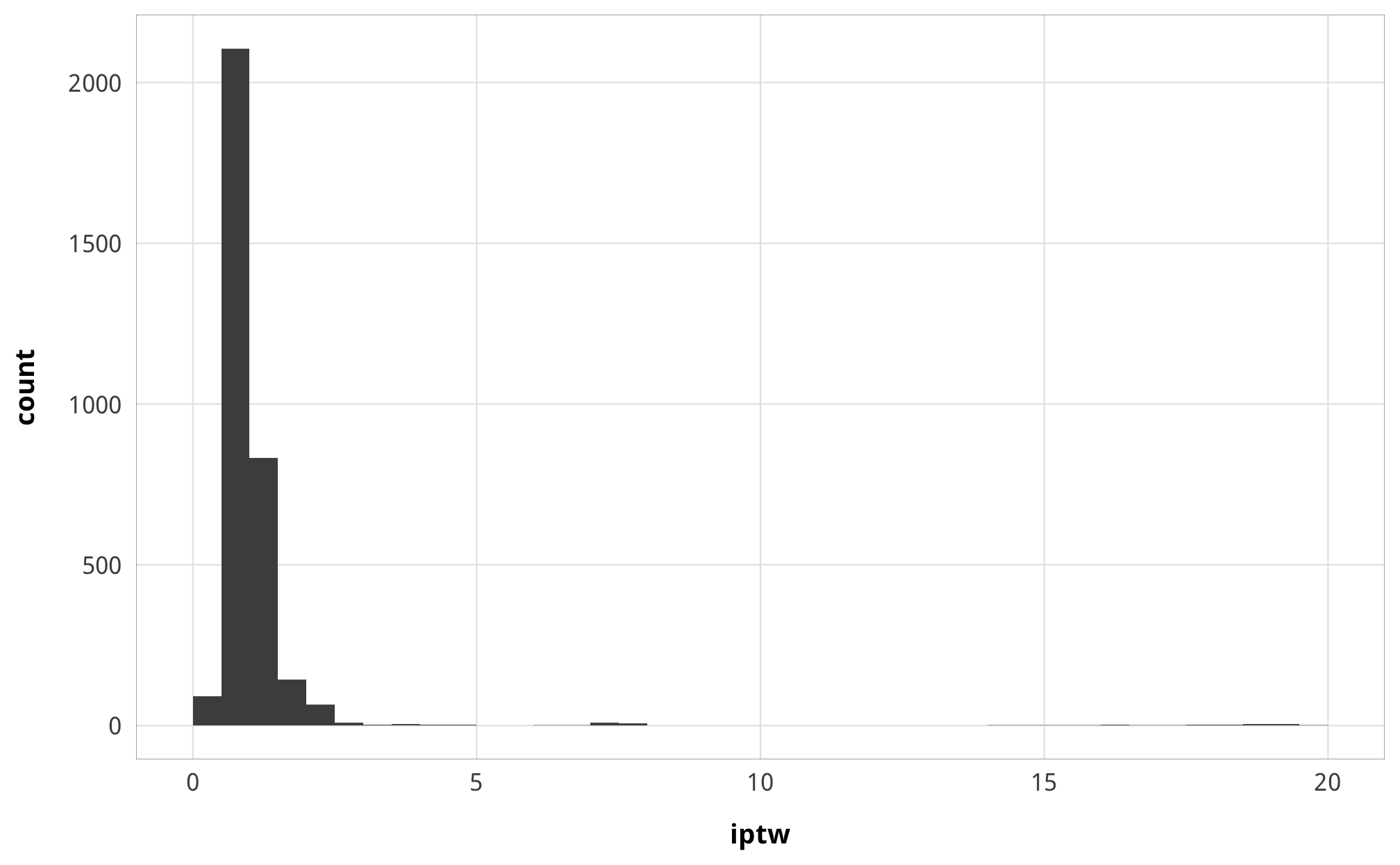#### Advocacy Great. summary(df_purpose_iptw_advocacy$iptw)
##    Min. 1st Qu.  Median    Mean 3rd Qu.    Max.
##  0.1081  0.9095  0.9759  1.0161  1.0214  7.0687
df_purpose_iptw_advocacy %>%
ggplot(aes(x = iptw)) +
geom_histogram(binwidth = 1, boundary = 0) +
theme_donors()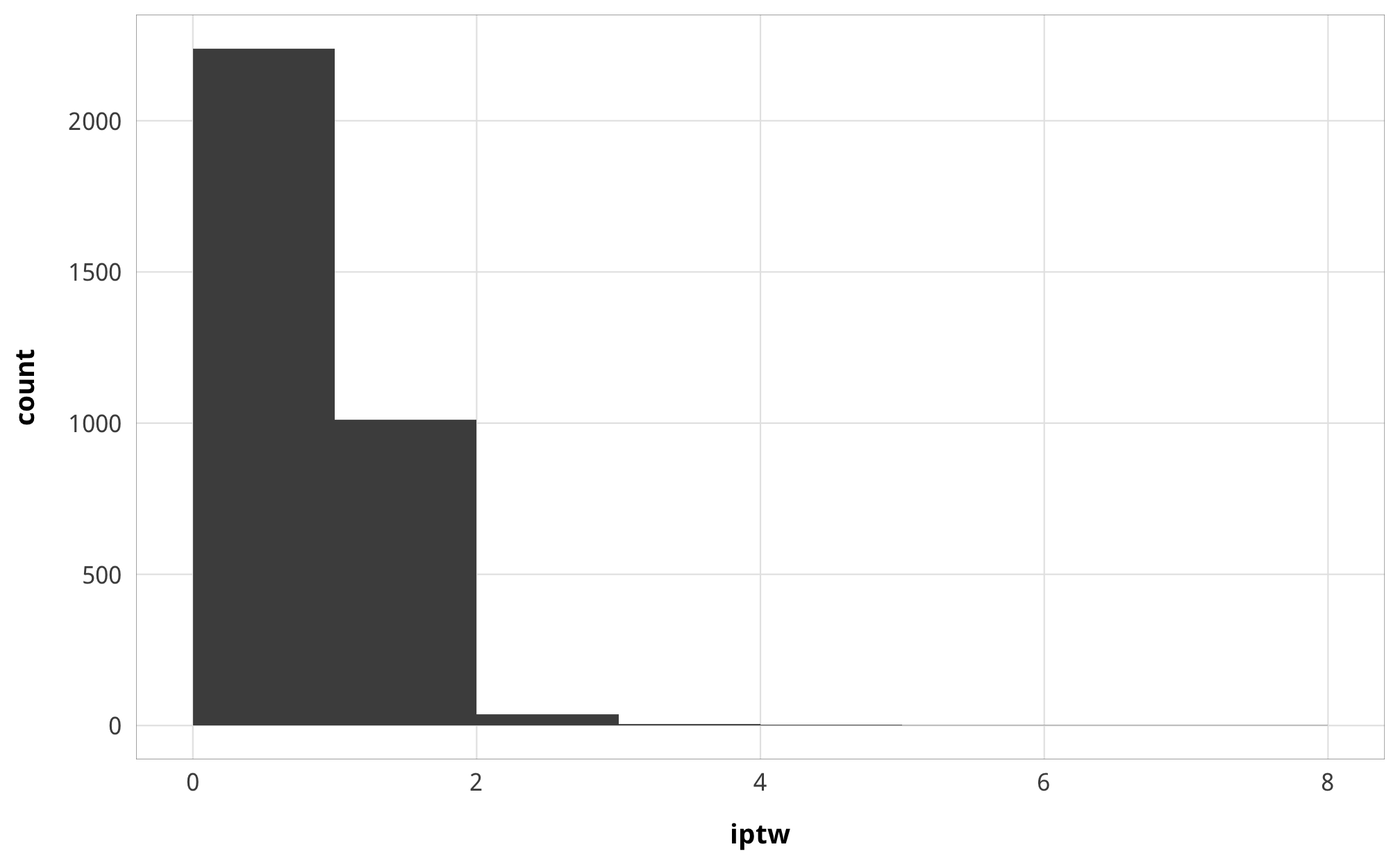Good.

summary(df_purpose_iptw_entry$iptw) ## Min. 1st Qu. Median Mean 3rd Qu. Max. ## 0.06705 0.90954 0.99114 1.02679 1.05879 5.80478 df_purpose_iptw_entry %>% ggplot(aes(x = iptw)) + geom_histogram(binwidth = 0.5, boundary = 0) + theme_donors()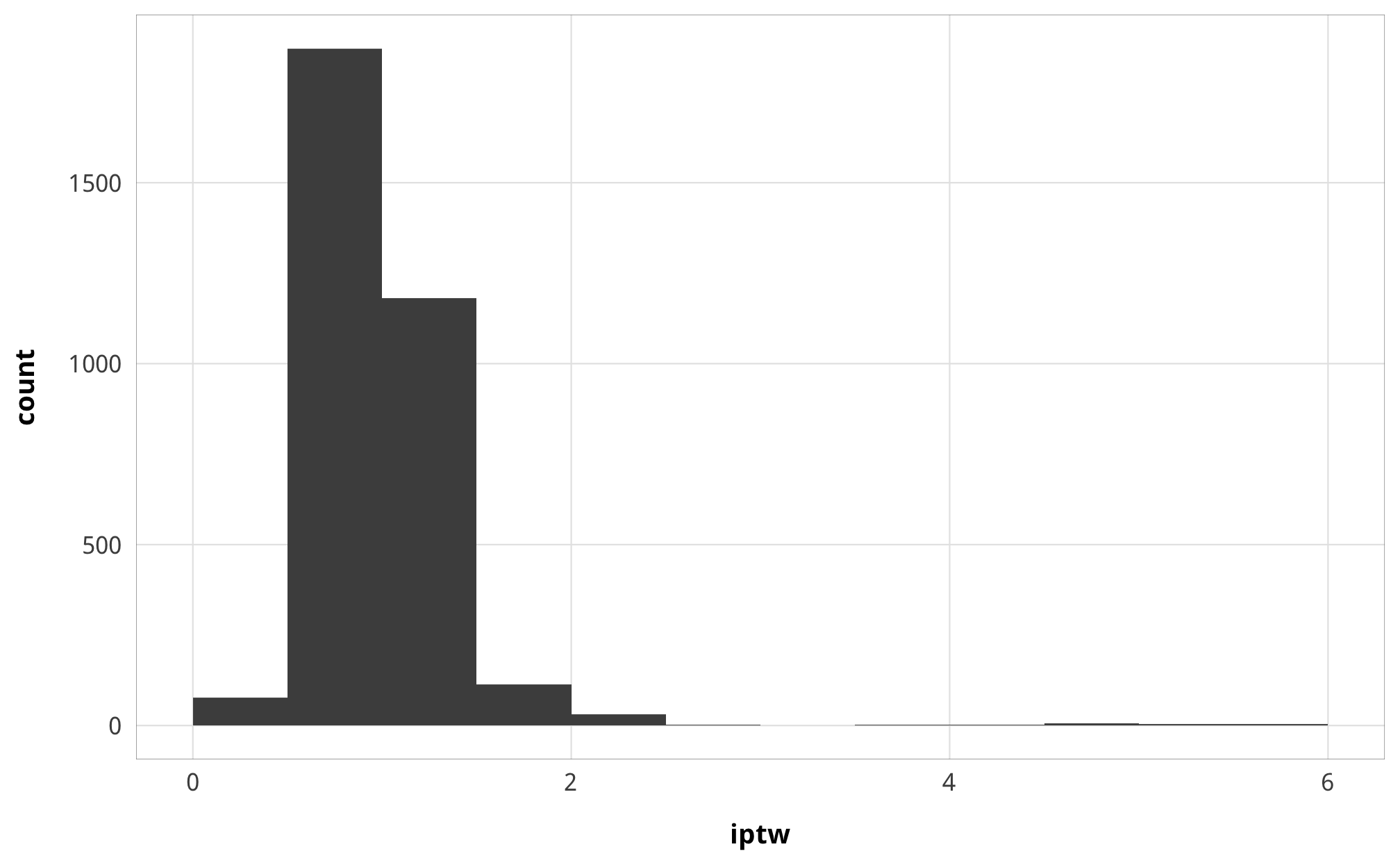#### Funding Some high values again, but only a handful, and the median and mean are great. summary(df_purpose_iptw_funding$iptw)
##     Min.  1st Qu.   Median     Mean  3rd Qu.     Max.
##  0.00198  0.85995  0.95694  1.09559  1.01442 22.28486
df_purpose_iptw_funding %>%
ggplot(aes(x = iptw)) +
geom_histogram(binwidth = 1, boundary = 0) +
theme_donors()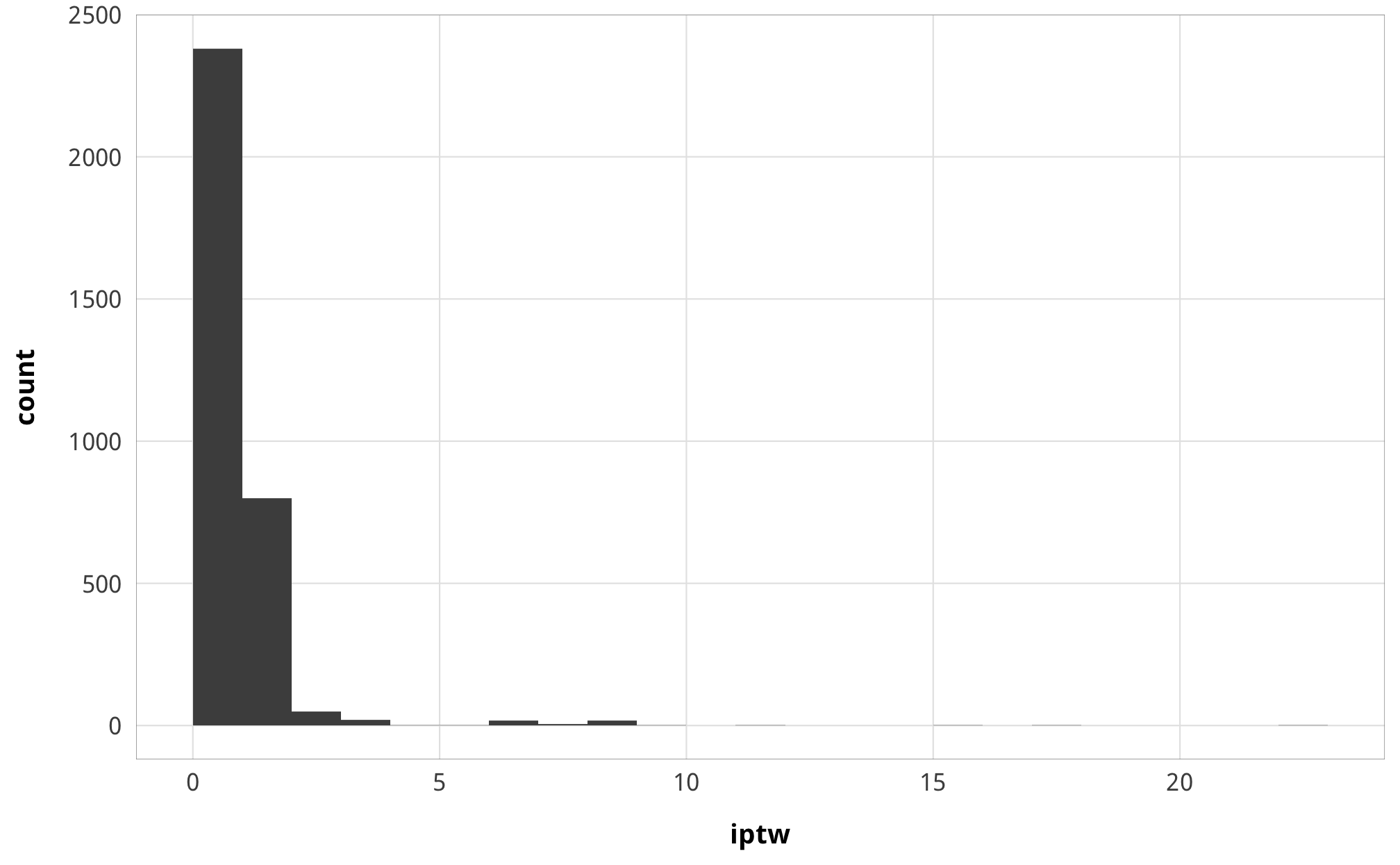hahaha oh no:

summary(df_purpose_iptw_ccsiiptw) ## Min. 1st Qu. Median Mean 3rd Qu. Max. ## 0.000e+00 0.000e+00 0.000e+00 5.692e+09 1.000e+00 1.922e+12 We truncate at four different thresholds: 100, 500, 1,000, and 5,000: plot_iptw_ccsi <- bind_rows( df_purpose_iptw_ccsi %>% mutate(threshold = 100, iptw = ifelse(iptw > 100, 100, iptw)), df_purpose_iptw_ccsi %>% mutate(threshold = 500, iptw = ifelse(iptw > 500, 500, iptw)), df_purpose_iptw_ccsi %>% mutate(threshold = 1000, iptw = ifelse(iptw > 1000, 1000, iptw)), df_purpose_iptw_ccsi %>% mutate(threshold = 5000, iptw = ifelse(iptw > 5000, 5000, iptw)) ) %>% mutate(threshold = paste0("Truncated at ", comma(threshold)), threshold = fct_inorder(threshold)) plot_iptw_ccsi %>% ggplot(aes(x = iptw)) + geom_histogram(bins = 50, boundary = 0) + facet_wrap(vars(threshold), scales = "free_x") + theme_donors()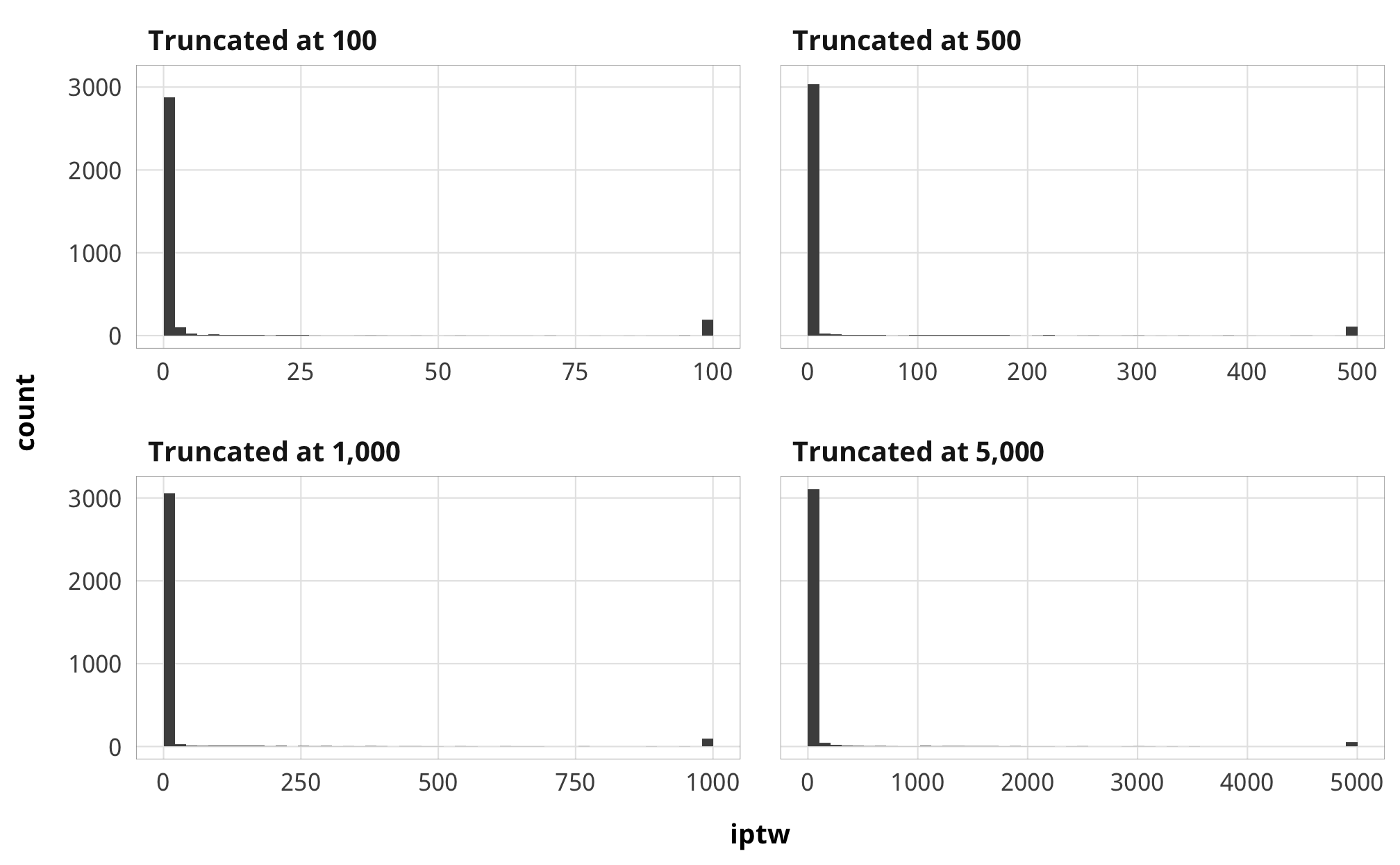### Results These are less important since we just use these treatment models to generate weights and because interpreting each coefficient when trying to isolate casual effects is unimportant and a “nuisance”. But here are the results anyway: #### Numerator models # model_names <- paste0("(", 1:length(models_tbl_h2_treatment_num), ")") model_names <- 1:length(models_tbl_h2_treatment_num) names(models_tbl_h2_treatment_num) <- model_names treatment_names <- c("Treatment", "Total barriers (t)", "Barriers to advocacy (t)", "Barriers to entry (t)", "Barriers to funding (t)", "CCSI (t)") treatment_rows <- as.data.frame(t(treatment_names)) attr(treatment_rows, "position") <- 1 coef_list_num <- list( "b_barriers_total_lag1" = "Treatment (t − 1)", "b_advocacy_lag1" = "Treatment (t − 1)", "b_entry_lag1" = "Treatment (t − 1)", "b_funding_lag1" = "Treatment (t − 1)", "b_v2xcs_ccsi_lag1" = "Treatment (t − 1)", "b_Intercept" = "Intercept" ) modelsummary(models_tbl_h2_treatment_num, statistic = "[{conf.low}, {conf.high}]", coef_map = coef_list_num, add_rows = treatment_rows, gof_omit = "ELPD", escape = FALSE, notes = list("Posterior means; 95% credible intervals in brackets")) 1 2 3 4 5 Treatment Total barriers (t) Barriers to advocacy (t) Barriers to entry (t) Barriers to funding (t) CCSI (t) Treatment (t − 1) 0.987 0.992 0.970 0.990 0.830 [0.977, 0.995] [0.983, 1.000] [0.958, 0.982] [0.979, 0.999] [0.810, 0.848] Intercept 0.086 0.015 0.051 0.030 0.112 [0.065, 0.108] [0.010, 0.020] [0.039, 0.063] [0.021, 0.039] [0.099, 0.126] Num.Obs. 3153 3153 3153 3153 3267 R2 0.941 0.949 0.929 0.940 0.959 R2 Marg. 0.941 0.949 0.928 0.940 0.912 LOOIC 4139.0 -4304.5 -12.6 -387.3 -9445.1 LOOIC s.e. 546.4 633.1 458.8 699.6 337.4 WAIC 4140.9 -4299.9 -12.4 -370.8 -9445.7 RMSE 1.33 0.34 0.66 0.60 0.33 Posterior means; 95% credible intervals in brackets #### Denominator models # model_names <- paste0("(", 1:length(models_tbl_h2_treatment_denom), ")") model_names <- 1:length(models_tbl_h2_treatment_denom) names(models_tbl_h2_treatment_denom) <- model_names treatment_names <- c("Treatment", "Total barriers (t)", "Barriers to advocacy (t)", "Barriers to entry (t)", "Barriers to funding (t)", "CCSI (t)") treatment_rows <- as.data.frame(t(treatment_names)) attr(treatment_rows, "position") <- 1 coef_list_denom <- list( "b_barriers_total_lag1" = "Treatment (t − 1)", "b_advocacy_lag1" = "Treatment (t − 1)", "b_entry_lag1" = "Treatment (t − 1)", "b_funding_lag1" = "Treatment (t − 1)", "b_v2xcs_ccsi_lag1" = "Treatment (t − 1)", "b_v2x_polyarchy" = "Polyarchy", "b_v2x_corr" = "Corruption index", "b_v2x_rule" = "Rule of law index", "b_v2x_civlib" = "Civil liberties index", "b_v2x_clphy" = "Physical violence index", "b_v2x_clpriv" = "Private civil liberties index", "b_gdpcap_log" = "Log GDP/capita", "b_un_trade_pct_gdp" = "Percent of GDP from trade", "b_v2peedueq" = "Educational equality index", "b_v2pehealth" = "Health equality index", "b_e_peinfmor" = "Infant mortality rate", "b_internal_conflict_past_5TRUE" = "Internal conflict in past 5 years", "b_natural_dis_count" = "Count of natural disasters", "b_Intercept" = "Intercept" ) modelsummary(models_tbl_h2_treatment_denom, statistic = "[{conf.low}, {conf.high}]", coef_map = coef_list_denom, add_rows = treatment_rows, gof_omit = "ELPD", escape = FALSE, notes = list("Posterior means; 95% credible intervals in brackets")) 1 2 3 4 5 Treatment Total barriers (t) Barriers to advocacy (t) Barriers to entry (t) Barriers to funding (t) CCSI (t) Treatment (t − 1) 0.974 0.986 0.964 0.977 0.504 [0.965, 0.985] [0.977, 0.995] [0.953, 0.975] [0.966, 0.987] [0.477, 0.530] Polyarchy -0.093 0.014 -0.078 -0.040 0.010 [-0.249, 0.083] [-0.033, 0.057] [-0.166, 0.018] [-0.128, 0.038] [-0.018, 0.040] Corruption index -0.066 -0.021 -0.023 -0.027 -0.020 [-0.234, 0.109] [-0.066, 0.022] [-0.109, 0.065] [-0.116, 0.052] [-0.054, 0.014] Rule of law index -0.107 -0.050 -0.027 -0.033 0.029 [-0.311, 0.083] [-0.100, 0.000] [-0.128, 0.071] [-0.122, 0.063] [-0.010, 0.069] Civil liberties index -0.386 -0.109 -0.177 -0.075 0.856 [-0.860, 0.125] [-0.244, 0.021] [-0.458, 0.085] [-0.325, 0.175] [0.775, 0.931] Physical violence index 0.233 0.062 0.113 0.052 -0.223 [0.010, 0.450] [0.002, 0.122] [-0.009, 0.235] [-0.056, 0.162] [-0.259, -0.185] Private civil liberties index 0.090 0.043 0.074 -0.033 -0.182 [-0.161, 0.370] [-0.026, 0.116] [-0.060, 0.222] [-0.172, 0.092] [-0.224, -0.141] Log GDP/capita -0.013 -0.002 -0.004 -0.007 -0.008 [-0.034, 0.008] [-0.008, 0.003] [-0.015, 0.008] [-0.017, 0.004] [-0.014, -0.002] Percent of GDP from trade -0.009 0.005 -0.011 -0.004 -0.005 [-0.053, 0.031] [-0.005, 0.016] [-0.033, 0.011] [-0.024, 0.016] [-0.013, 0.003] Educational equality index -0.011 -0.001 -0.002 -0.009 -0.007 [-0.038, 0.016] [-0.008, 0.006] [-0.015, 0.013] [-0.022, 0.004] [-0.013, -0.002] Health equality index 0.015 0.001 0.000 0.014 0.000 [-0.015, 0.048] [-0.008, 0.009] [-0.016, 0.016] [0.000, 0.031] [-0.006, 0.006] Infant mortality rate -0.001 0.000 0.000 0.000 0.000 [-0.002, 0.000] [0.000, 0.000] [-0.001, 0.000] [-0.001, 0.000] [0.000, 0.000] Internal conflict in past 5 years 0.006 0.004 0.002 0.002 0.008 [-0.035, 0.048] [-0.006, 0.015] [-0.021, 0.023] [-0.019, 0.022] [0.002, 0.013] Count of natural disasters 0.006 0.001 0.002 0.002 0.000 [0.001, 0.011] [0.000, 0.003] [0.000, 0.005] [0.000, 0.005] [-0.001, 0.001] Intercept 0.434 0.068 0.165 0.201 0.084 [0.191, 0.685] [0.007, 0.131] [0.035, 0.289] [0.079, 0.323] [0.025, 0.146] Num.Obs. 3153 3153 3153 3153 3267 R2 0.942 0.949 0.929 0.941 0.970 R2 Marg. 0.942 0.949 0.928 0.941 0.948 LOOIC 4121.4 -4296.8 -16.1 -398.7 -10406.1 LOOIC s.e. 535.6 630.7 454.2 685.4 269.7 WAIC 4125.7 -4291.5 -17.5 -382.0 -10407.1 RMSE 1.33 0.34 0.66 0.60 0.33 Posterior means; 95% credible intervals in brackets ## Outcome models ### Modeling choice Our dependent variable for this hypothesis is the percentage of ODA allocated for contentious purposes, again leaded by one year. We classify contentious aid as any project focused on government and civil society (DAC codes 150 and 151) or conflict prevention and resolution, peace and security (DAC code 152). Working with proportion data, however, poses interesting mathematical and methodological challenges, since the range of possible outcomes is limited to a value between 0 and 1. Treating proportion variables in a mixed model is technically possible, but it yields predictions that go beyond the allowable range of values (1.13, -0.5, etc.). Treating the proportion as a binomial variable is also possible and is indeed one of the ways to use the glm() function in R. However, this entails considering the proportion as a ratio of success and failures. In this case, treating a dollar of contentious aid as a success feels off, especially since aid amounts aren’t independent events—it’s not like each dollar of aid goes through a probabalistic process like a coin flip. Another solution is to use beta regression, which constrains the outcome variable to values between 0 and 1, but unfortunately does not allow for values of exactly 0 or 1. Zero-and-one inflated beta regression models, however, make adjustments for this and model the probability of being 0, being 1, and being somewhere in the middle using different processes. Matti Vuorre has an excellent overview of ZOIB models here. However, as we found with our hurdling dilemma with H1, while it’s possible to fit a zero-inflated model (or even zero-one-inflated model), it would require separate model specifications for different parts (the zero part, the one part, and the non-zero-one parts), leaving us with three different pseudo-treatment effects, only one of which (the non-zero-one part) weighted with the IPTW, and thus adjusted with do-calculus logic. One recommendation by Ben Bolker, the maintainer of lme4, is to use a logit transformation of the dependent variable in lmer() models. This seems to be standard practice in political science research, too. Logit transformations still can’t handle values of exactly 0 or 1, though, but it’s possible to winsorize those values by adding or subtracting 0.001 to the extremes. Stata has a guide about how to do this too. We thus use logit-linear models of the ratio of contentious aid to non-contentious aid, like this: $\log \left( \frac{\text{Contentious aid}}{\text{Noncontentious aid}} \right)_{i, t+1}$ That means that we’re comparing the ratio of contentious aid to non-contentious aid rather than the direct percent of contentious aid, and it makes for some acrobatic interpretations, but at least the results are interpretable. ### Interpreting outcome models TODO ### Priors for outcome models Here’s the full specification for the models and all priors: \begin{aligned} \log \left(\frac{\text{Contentious aid}}{\text{Noncontentious aid}} \right)_{i, t} &\sim \text{IPTW}_{i, t-1} \times \mathcal{N} (\mu_{i, t}, \sigma_m) & \text{[likelihood]}\\ \mu_{i, t} &\sim \alpha_{\text{country}[i]} + \delta_{\text{year}[k]} + \beta\ \text{Law}_{i, t-1} & \text{[marginal structural model]}\\ \alpha_j &\sim \mathcal{N} (\bar{\alpha}, \sigma_{\alpha}) \text{, for } j \text{ in } 1 .. J & \text{[country-specific intercepts]} \\ \delta_j &\sim \mathcal{N} (0, \sigma_{\delta}) \text{, for } j \text{ in } 1 .. J & \text{[year-specific intercepts]} \\ \ \\ \bar{\alpha} &\sim \mathcal{N} (0, 20) & \text{[prior population intercept]} \\ \beta &\sim \mathcal{N} (0, 3) & \text{[prior population effects]} \\ \sigma_m, \sigma_{\alpha}, \sigma_{\delta} &\sim \operatorname{Cauchy}(0, 1) & \text{[prior sd for errors]} \end{aligned} ### Check outcome models The traceplots all look fine. Here’s one as an example: m_purpose_outcome_total %>% posterior_samples(add_chain = TRUE) %>% select(-starts_with("r_gwcode"), -starts_with("z_"), -starts_with("r_year"), -lp__, -iter) %>% mcmc_trace() + theme_donors()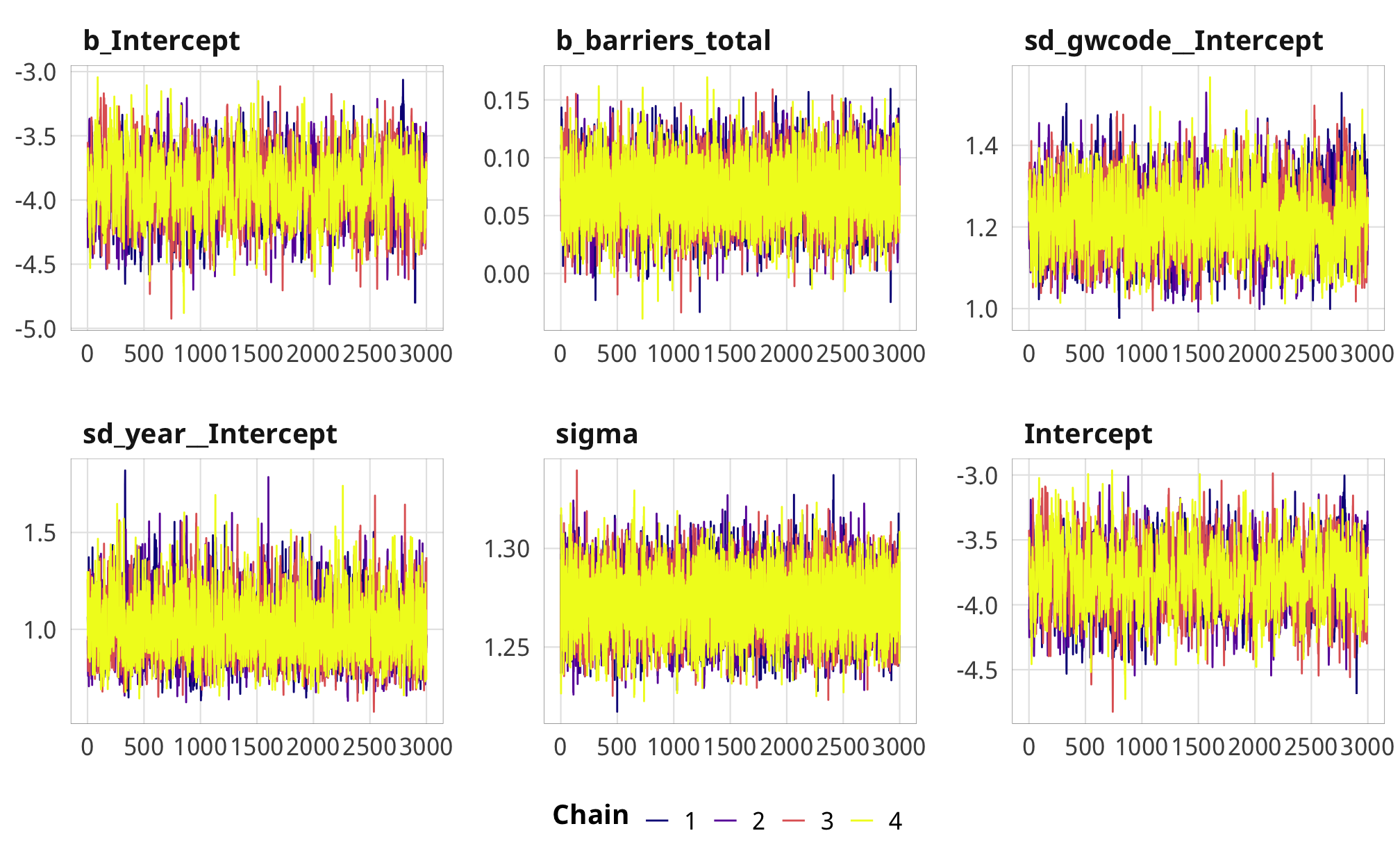### Results #### ATE plots coefs_nice <- tribble( ~.variable, ~var_nice, "b_barriers_total", "Additional law, t - 1", "b_advocacy", "Additional advocacy law, t - 1", "b_entry", "Additional entry law, t - 1", "b_funding", "Additional funding law, t - 1", "b_v2xcs_ccsi", "Core civil society index, t - 1" ) %>% mutate(var_nice = fct_inorder(var_nice)) plot_total_data <- gather_draws(m_purpose_outcome_total, b_barriers_total) plot_advocacy_data <- gather_draws(m_purpose_outcome_advocacy, b_advocacy) plot_entry_data <- gather_draws(m_purpose_outcome_entry, b_entry) plot_funding_data <- gather_draws(m_purpose_outcome_funding, b_funding) plot_purpose_coefs <- bind_rows(plot_total_data, plot_advocacy_data, plot_entry_data, plot_funding_data) %>% left_join(coefs_nice, by = ".variable") %>% mutate(.value = exp(.value)) rope_sd <- exp(sd(df_purpose_iptw_totalprop_contentious_logit_lead1))

plot_rope <- tibble(xmin = 1 + (-0.05 * rope_sd),
xmax = 1 + (0.05 * rope_sd))

ggplot(plot_purpose_coefs, aes(x = .value, y = fct_rev(var_nice), fill = var_nice)) +
geom_vline(xintercept = 1) +
geom_rect(data = plot_rope, aes(xmin = xmin, xmax = xmax, ymin = -Inf, ymax = Inf),
fill = "#FFDC00", alpha = 0.3, inherit.aes = FALSE) +
annotate(geom = "label", x = 1, y = 0.65,
label = "Region of practical equivalence\n(± 0.05 × SD y)",
size = pts(8)) +
stat_halfeye(.width = c(0.8, 0.95), alpha = 0.8, point_interval = "median_hdi") +
guides(fill = FALSE) +
scale_y_discrete() +
scale_fill_manual(values = c("#FF851B", "#85144b", "#3D9970", "#001f3f")) +
labs(x = "Percent change in ratio of contentious aid in following year", y = NULL,
caption = "Point shows posterior median; lines show 80% and 95%\ncredible highest-density-interval") +
theme_donors()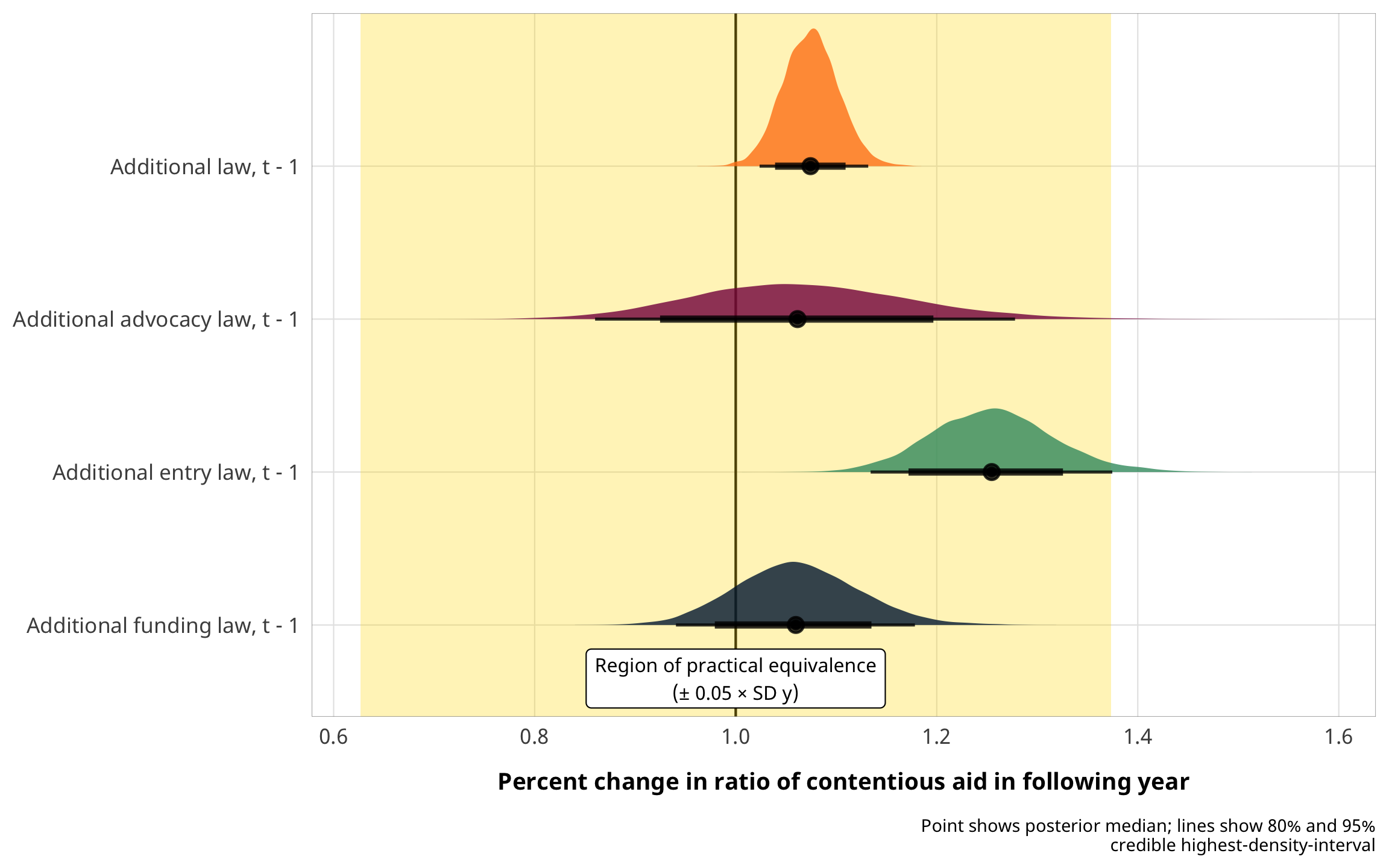lol what even

plot_ccsi_coefs <- m_purpose_outcome_ccsi %>%
map_dfr(~gather_draws(., b_v2xcs_ccsi), .id = "model") %>%
mutate(.value = .value / 10) %>%
mutate(model = comma(as.numeric(str_remove(model, "model_")))) %>%
mutate(model = fct_inorder(model)) %>%
left_join(coefs_nice, by = ".variable")

ggplot(plot_ccsi_coefs, aes(x = .value, y = fct_rev(model), fill = model)) +
stat_halfeye(.width = c(0.8, 0.95), point_interval = "median_hdi",
position = position_dodge(width = 0.5)) +
guides(fill = FALSE) +
labs(x = "Coefficient", y = "IPTW truncation point") +
theme_donors()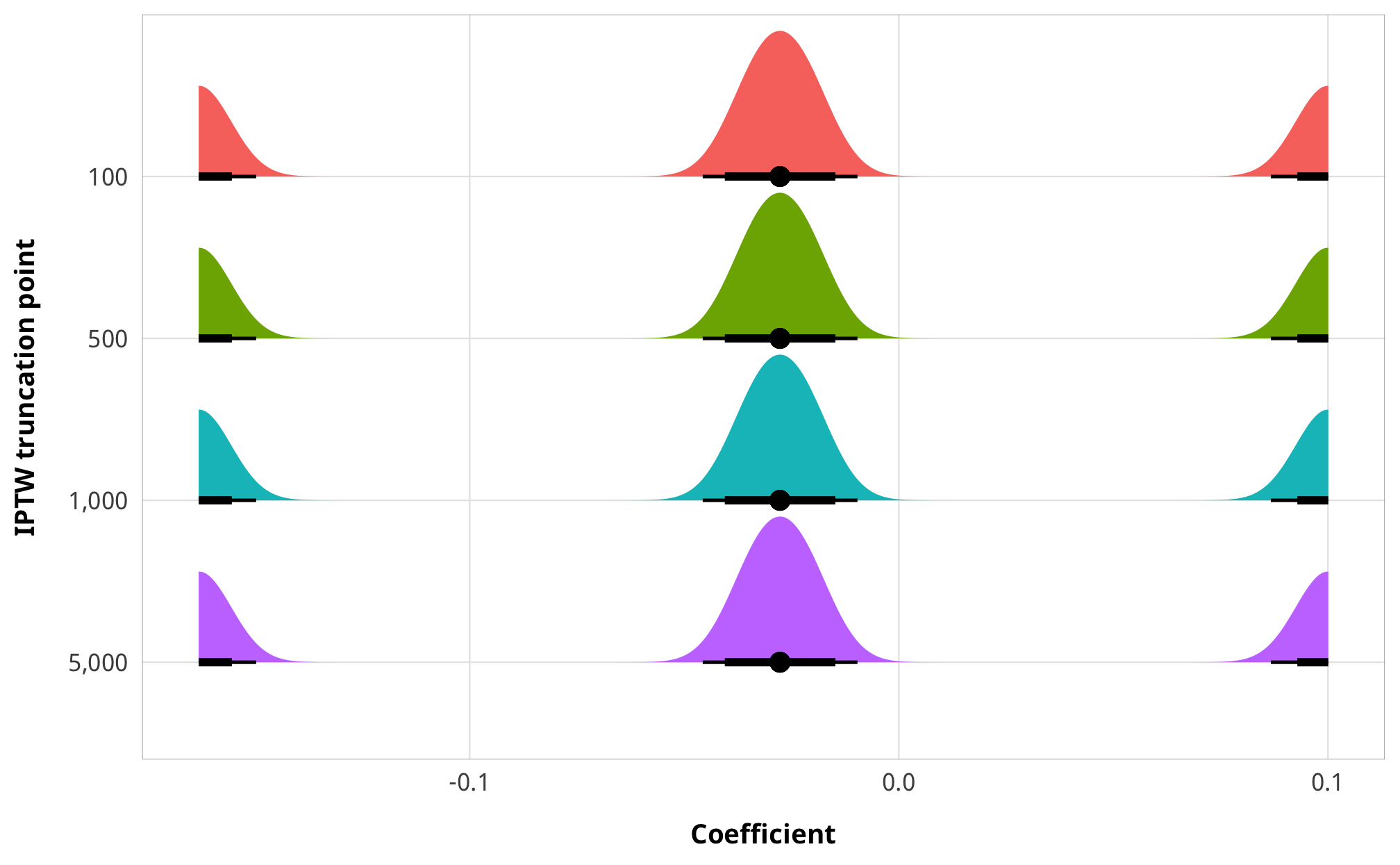#### Tables

# model_names <- paste0("(", 1:length(models_tbl_h2_outcome_dejure), ")")
model_names <- 1:length(models_tbl_h2_outcome_dejure)
names(models_tbl_h2_outcome_dejure) <- model_names

treatment_names <- c("Treatment", "Total barriers (t)", "Barriers to advocacy (t)",
"Barriers to entry (t)", "Barriers to funding (t)")
treatment_rows <- as.data.frame(t(treatment_names))
attr(treatment_rows, "position") <- 1

coef_list_outcome <- list(
"b_barriers_total" = "Treatment (t)",
"b_entry" = "Treatment (t)",
"b_funding" = "Treatment (t)",
"b_v2xcs_ccsi" = "Core civil society index (t)",
"b_Intercept" = "Intercept",
"sd_gwcode__Intercept" = "σ country intercepts",
"sd_year__Intercept" = "σ year intercepts"
)

modelsummary(models_tbl_h2_outcome_dejure,
statistic = "[{conf.low}, {conf.high}]",
coef_map = coef_list_outcome,
gof_omit = "ELPD",
escape = FALSE,
notes = list("Posterior means; 95% credible intervals in brackets")) %>%
add_header_above(c(" " = 1, "Outcome: Logit prop contentious (t + 1)" = 4))
Outcome: Logit prop contentious (t + 1)
1 2 3 4
Treatment Total barriers (t) Barriers to advocacy (t) Barriers to entry (t) Barriers to funding (t)
Treatment (t) 0.072 0.060 0.227 0.058
[0.023, 0.124] [-0.141, 0.252] [0.132, 0.323] [-0.053, 0.171]
Intercept -3.904 -3.810 -3.976 -3.840
[-4.355, -3.429] [-4.254, -3.363] [-4.426, -3.525] [-4.320, -3.382]
σ country intercepts 1.216 1.194 1.192 1.197
[1.078, 1.377] [1.052, 1.354] [1.049, 1.347] [1.053, 1.349]
σ year intercepts 0.978 0.962 0.965 0.960
[0.741, 1.311] [0.711, 1.279] [0.705, 1.293] [0.711, 1.288]
Num.Obs. 3293 3293 3293 3293
R2 0.548 0.542 0.546 0.541
R2 Marg. 0.005 0.000 0.010 0.001
LOOIC 12772.0 11593.3 11726.7 12330.7
LOOIC s.e. 274.2 145.5 173.9 213.9
WAIC 12769.7 11592.3 11725.5 12328.7
Posterior means; 95% credible intervals in brackets

TODO: This is a mess

truncation_thresholds <- names(models_tbl_h2_outcome_defacto) %>%
str_extract("_\\d+") %>%
str_remove("_") %>%
as.numeric() %>%
comma()

truncation_names <- c("IPTW truncation threshold", truncation_thresholds)
truncation_rows <- as.data.frame(t(truncation_names))
attr(truncation_rows, "position") <- 1

# model_names <- paste0("(", 1:length(models_tbl_h2_outcome_defacto), ")")
model_names <- 1:length(models_tbl_h2_outcome_defacto)
names(models_tbl_h2_outcome_defacto) <- model_names

modelsummary(models_tbl_h2_outcome_defacto,
statistic = "[{conf.low}, {conf.high}]",
coef_map = coef_list_outcome,
gof_omit = "ELPD",
escape = FALSE,
notes = list("Posterior means; 95% credible intervals in brackets")) %>%
add_header_above(c(" " = 1, "Outcome: Outcome: Logit prop contentious (t + 1)" = 4))
Outcome: Outcome: Logit prop contentious (t + 1)
1 2 3 4
IPTW truncation threshold 100 500 1,000 5,000
Core civil society index (t) -0.277 -0.277 -0.277 -0.277
[-1.631, 1.001] [-1.631, 1.001] [-1.631, 1.001] [-1.631, 1.001]
Intercept 0.283 0.283 0.283 0.283
[-1.968, 2.757] [-1.968, 2.757] [-1.968, 2.757] [-1.968, 2.757]
Num.Obs. 3293 3293 3293 3293
R2 0.010 0.010 0.010 0.010
LOOIC 1.077603e+14 1.077603e+14 1.077603e+14 1.077603e+14
LOOIC s.e. 2.971661e+13 2.971661e+13 2.971661e+13 2.971661e+13
WAIC 1.904286e+25 1.904286e+25 1.904286e+25 1.904286e+25
Posterior means; 95% credible intervals in brackets

#### Marginal effects

# brms::conditional_effects() can create spaghetti plots (single lines per
# sample), but it stores them in an attribute slot named "spaghetti", which is
# tricky to extract data from. This pulls the data frame from the attribute
make_spaghetti <- function(model) {
raw_effects <- conditional_effects(model, spaghetti = TRUE, nsamples = 100)[]
spaghetti_data <- attr(raw_effects, "spaghetti")
return(spaghetti_data)
}

inv_logit <- function(f, a) {
a <- (1 - 2 * a)
(a * (1 + exp(f)) + (exp(f) - 1)) / (2 * a * (1 + exp(f)))
}

make_spaghetti(m_purpose_outcome_total) %>%
mutate(estimate__ = inv_logit(estimate__, a = 0.001)) %>%
ggplot(aes(x = effect1__, y = estimate__, group = sample__)) +
geom_line(size = 0.25, color = "#FF4136") +
scale_x_continuous(breaks = 0:9) +
scale_y_continuous(labels = percent_format(accuracy = 1)) +
guides(color = FALSE) +
labs(x = "Count of total legal barriers",
y = "Predicted proportion of\ncontentious aid in following year") +
theme_donors() +
theme(panel.grid.major.x = element_blank())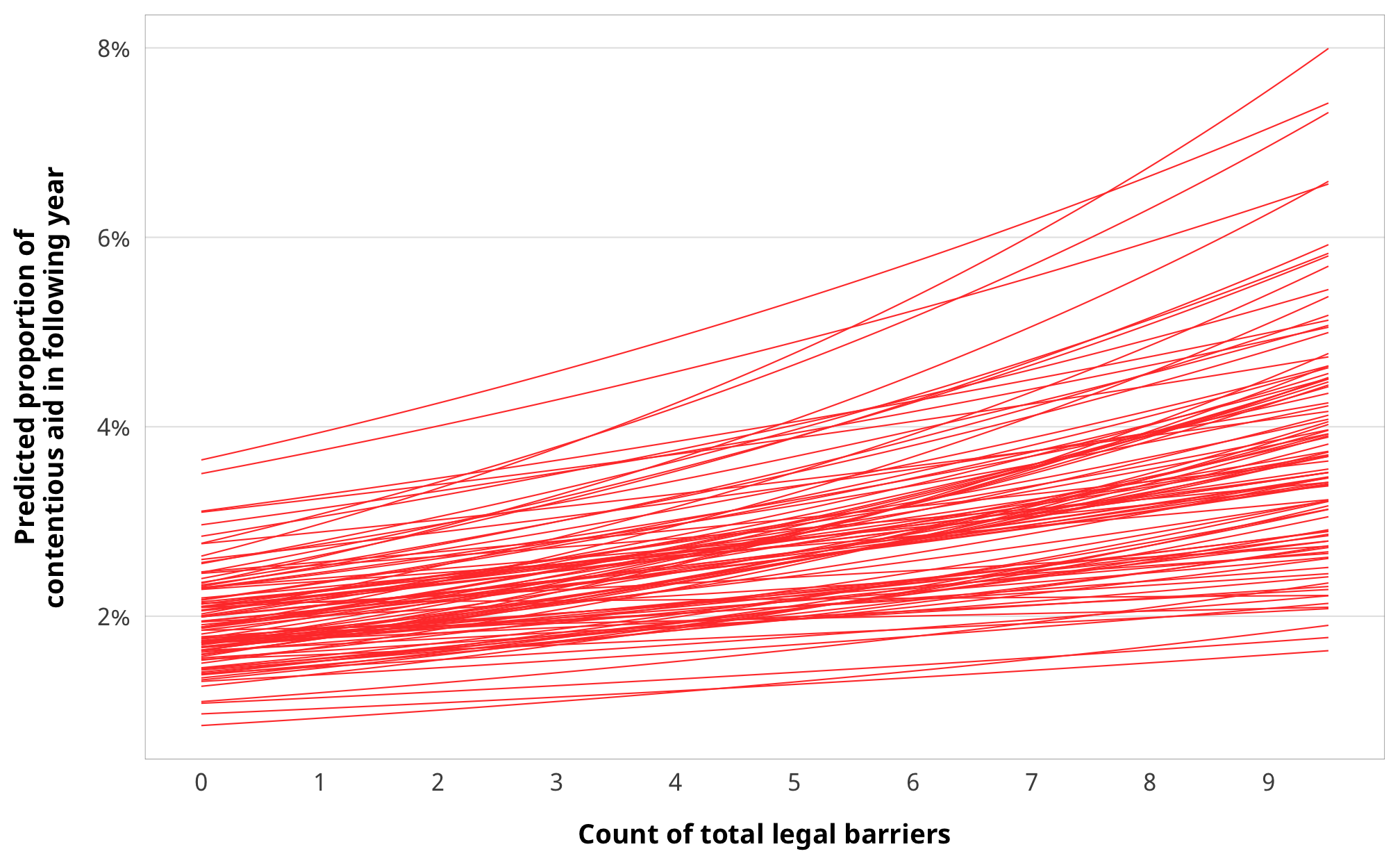make_spaghetti(m_purpose_outcome_advocacy) %>%
mutate(estimate__ = inv_logit(estimate__, a = 0.001)) %>%
ggplot(aes(x = effect1__, y = estimate__, group = sample__)) +
geom_line(size = 0.25, color = "#FF4136") +
scale_x_continuous(breaks = 0:2) +
scale_y_continuous(labels = percent_format(accuracy = 1)) +
guides(color = FALSE) +
labs(x = "Count of barriers to advocacy",
y = "Predicted proportion of\ncontentious aid in following year") +
theme_donors() +
theme(panel.grid.major.x = element_blank())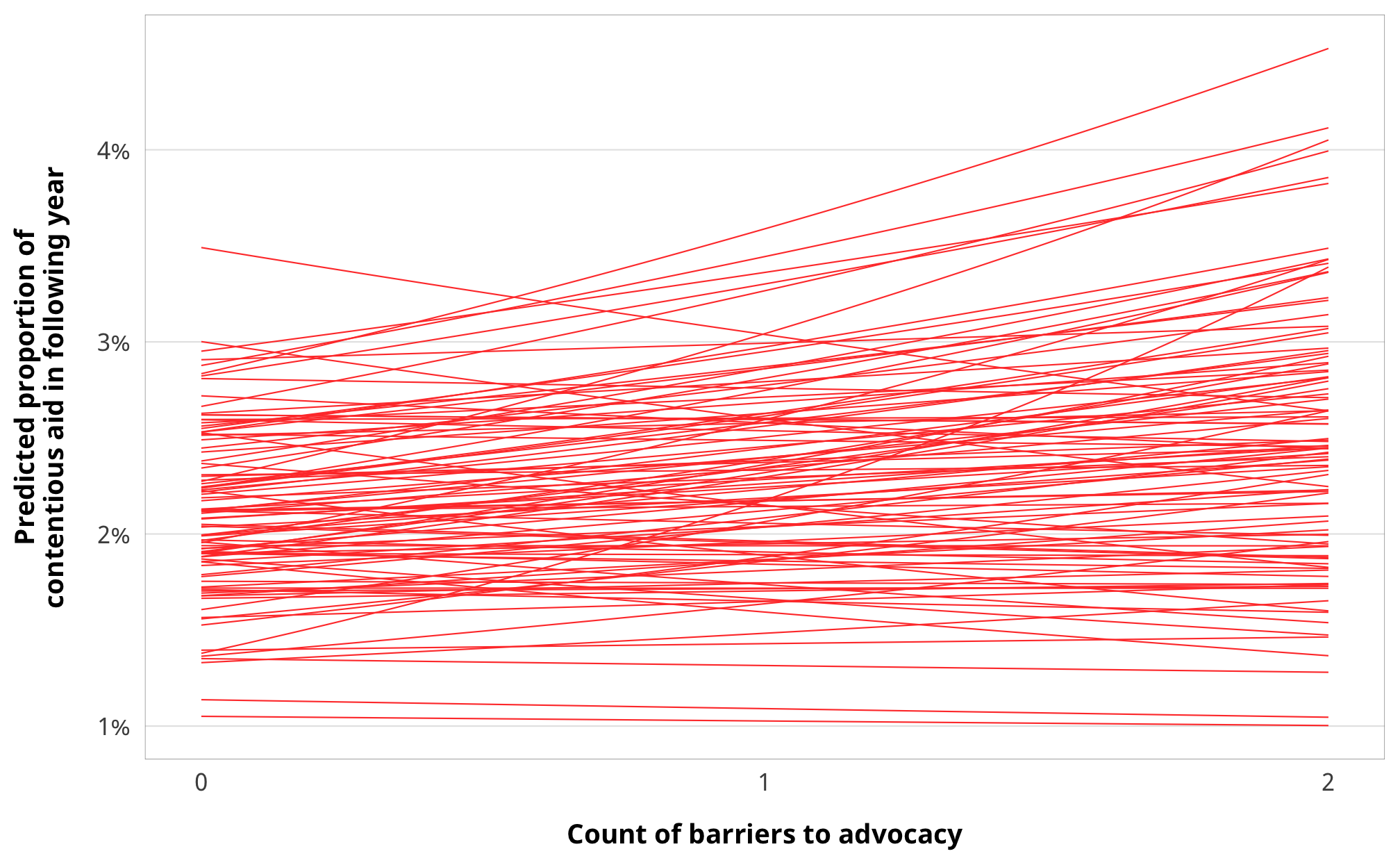make_spaghetti(m_purpose_outcome_entry) %>%
mutate(estimate__ = inv_logit(estimate__, a = 0.001)) %>%
ggplot(aes(x = effect1__, y = estimate__, group = sample__)) +
geom_line(size = 0.25, color = "#FF4136") +
scale_x_continuous(breaks = 0:3) +
scale_y_continuous(labels = percent_format(accuracy = 1)) +
guides(color = FALSE) +
labs(x = "Count of barriers to entry",
y = "Predicted proportion of\ncontentious aid in following year") +
theme_donors() +
theme(panel.grid.major.x = element_blank())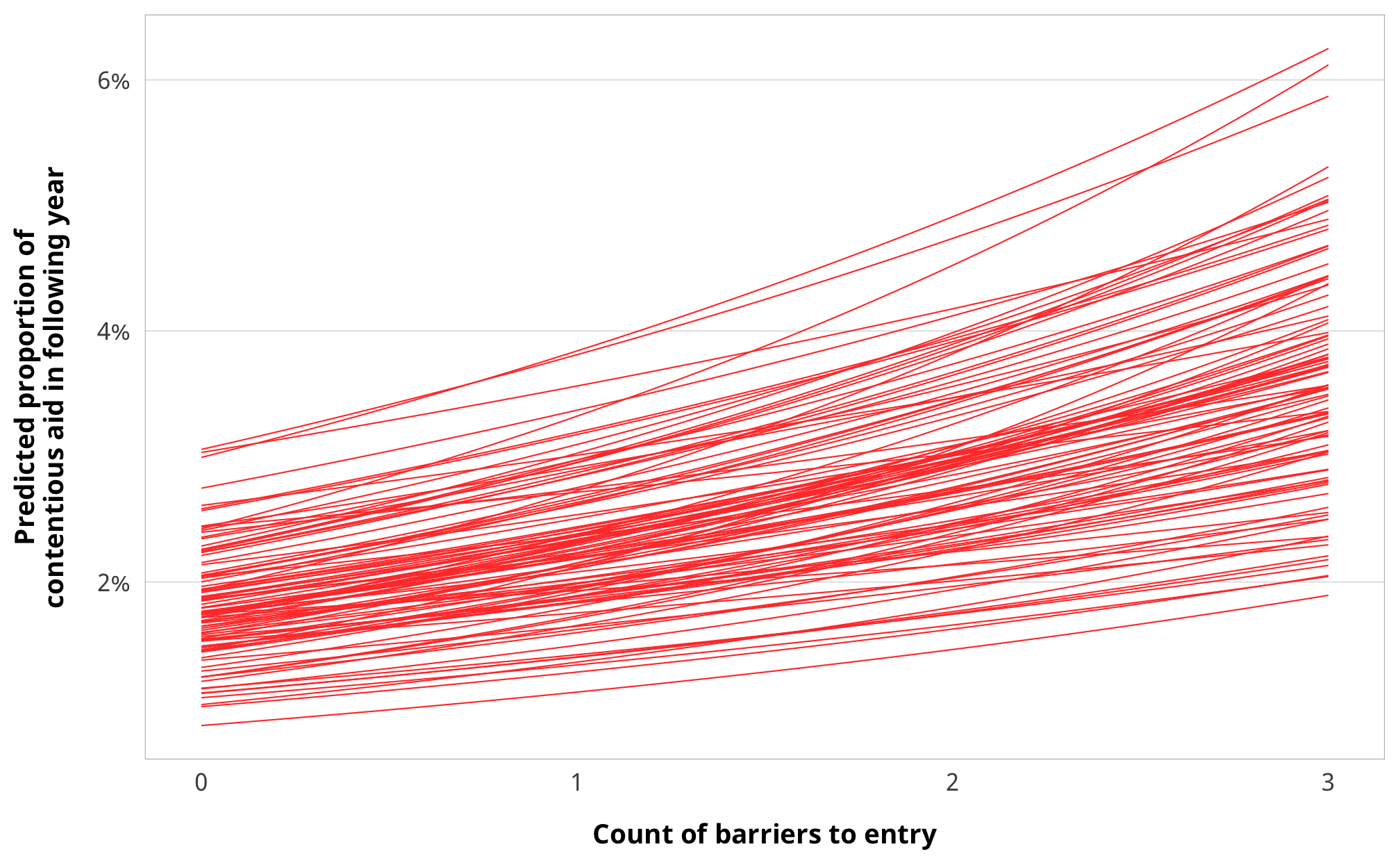make_spaghetti(m_purpose_outcome_funding) %>%
mutate(estimate__ = inv_logit(estimate__, a = 0.001)) %>%
ggplot(aes(x = effect1__, y = estimate__, group = sample__)) +
geom_line(size = 0.25, color = "#FF4136") +
scale_x_continuous(breaks = 0:5) +
scale_y_continuous(labels = percent_format(accuracy = 1)) +
guides(color = FALSE) +
labs(x = "Count of barriers to funding",
y = "Predicted proportion of\ncontentious aid in following year") +
theme_donors() +
theme(panel.grid.major.x = element_blank())